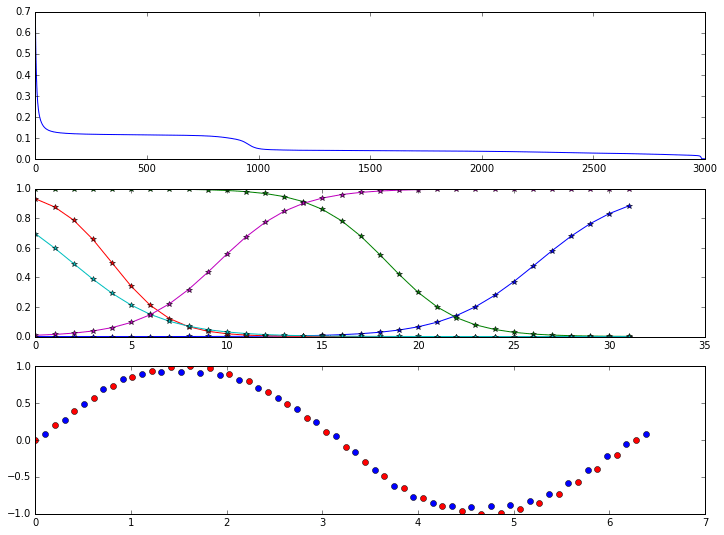# A Neural Network in Python, Part 2: activation functions, bias, SGD, etc.This is Part 2 of A Neural Network in Python, which was a very simple neural network to learn the XOR function. This part builds on that example to demonstrate more activation functions, learning a simple math function, adding a bias, improvements to the initial random weights, stochastic gradient descent, mean square error loss function,…

# A Neural Network in Python, Part 1: sigmoid function, gradient descent & backpropagationIn this article, I’ll show you a toy example to learn the XOR logical function. My objective is to make it as easy as possible for you to to see how the basic ideas work, and to provide a basis from which you can experiment further. In real applications, you would not write these programs from scratch…